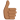# What conditions are needed to get a stable limit cycle here?

## Homework Statement

I want to find conditions over A,B,C,D to observe a stable limit cycle in the following system:
$$\frac{dx}{dt} = x \; (A-B y) \hspace{1cm} \frac{dy}{dt} = -y \; (C-D x)$$

## Homework Equations

Setting dx/dt=0 and dy/dt=0 you can find that (C/D , A/B) is a fixpoint.

## The Attempt at a Solution

When I evaluate the Jacobian matrix in that point...
$$J(x,y) = \left[ \begin{matrix} A-B y & -B x \\ D y & D x - C \end{matrix} \right] \: \Rightarrow \: J\left(\frac{C}{D}, \frac{A}{B}\right) = \left[ \begin{matrix} 0 & -\frac{BC}{D} \\ \frac{DA}{B} & 0 \end{matrix} \right]$$
Since λ1 = λ2 = 0, a Hopf bifurcation could occur (If and only if Tr(J)=0 ∧ Det(J)>0). As a consequence... it's necesary to have A,B,C,D such that AC > 0, however, by definition A,B,C,D are positive.

Does it mean that I will always have a bifurcation? If so, why am I getting stable and unstable orbits when I change the parameters?. Also, if this approach is not useful to determine the requirements to have a stable limit cycle, then, what could be the best approach? (Sorry if these questions sound kinda basic - I'm trying to learn about this topic by myself)

Related Calculus and Beyond Homework Help News on Phys.org
Thanks for the post! This is an automated courtesy bump. Sorry you aren't generating responses at the moment. Do you have any further information, come to any new conclusions or is it possible to reword the post?

epenguin
Homework Helper
Gold Member
This does not look like a system with a limit cycle, it looks like a Volterra-Lotka system, where you just have an infinite number of concentric cycles.

it looks like a Volterra-Lotka system
You're right, however I'll have to search a lot more about this topic. If you know any book that would be helpful, I'd appreciate it.

By the way, I said I was "getting stable orbits" but... well, I wasn't studying the system for a sufficient long period of time so, yeah, it's just like you already said it... concentric cycles but nothing like a stable orbit. Thanks for the commentepenguin
Homework Helper
Gold Member
At the moment I have forgotten stuff I knew even not that long ago, but I think with a small modification of this Volterra-Lotka system you can get limit cycles.

Others can suggest books, but you probably want fairly introductory or elementary. If your orientation is engineering, there is "nonlinear ordinary differential equations" by Jordan and Smith. Instead for biology, the most elementary if you can find it and written for biologists with little maths background is "modelling dynamic phenomena in molecular and cellular biology" by L A Segel. (1984). To read these books is to realise you could have worked most of it out yourself. Segel afterwards produced a number of books I have not seen, but which seem to be similar but taking it further with titles like "A primer on mathematical models in biology"

•JuanC97
pasmith
Homework Helper

## Homework Statement

I want to find conditions over A,B,C,D to observe a stable limit cycle in the following system:
$$\frac{dx}{dt} = x \; (A-B y) \hspace{1cm} \frac{dy}{dt} = -y \; (C-D x)$$

## Homework Equations

Setting dx/dt=0 and dy/dt=0 you can find that (C/D , A/B) is a fixpoint.

## The Attempt at a Solution

When I evaluate the Jacobian matrix in that point...
$$J(x,y) = \left[ \begin{matrix} A-B y & -B x \\ D y & D x - C \end{matrix} \right] \: \Rightarrow \: J\left(\frac{C}{D}, \frac{A}{B}\right) = \left[ \begin{matrix} 0 & -\frac{BC}{D} \\ \frac{DA}{B} & 0 \end{matrix} \right]$$
Since λ1 = λ2 = 0, a Hopf bifurcation could occur (If and only if Tr(J)=0 ∧ Det(J)>0). As a consequence... it's necesary to have A,B,C,D such that AC > 0, however, by definition A,B,C,D are positive.

Does it mean that I will always have a bifurcation? If so, why am I getting stable and unstable orbits when I change the parameters?. Also, if this approach is not useful to determine the requirements to have a stable limit cycle, then, what could be the best approach? (Sorry if these questions sound kinda basic - I'm trying to learn about this topic by myself)
The only bifurcations occur where $AC = 0$.

What happens when $AC = 0$ is that the fixed point at $(C/D, A/B)$ merges with the trivial fixed point at $(0,0)$. This is not a Hopf bifurcation; it's something more complicated. When $AC = 0$ you end up with one or both of the coordinate axes consisting of degenerate fixed points.

A Hopf bifurcation occurs where a pair of complex conjugate eigenvalues crosses the imaginary axis, so that the real part changes sign. That's not what's happening here; it's just not possible to vary the parameters to make the real parts of the eigenvalues of the Jacobian at $(C/D, A/B)$ anything other than zero. For all $AC > 0$ this fixed point is a center; varying $B$ or $D$ just changes the location of the fixed point, not its type.

•JuanC97